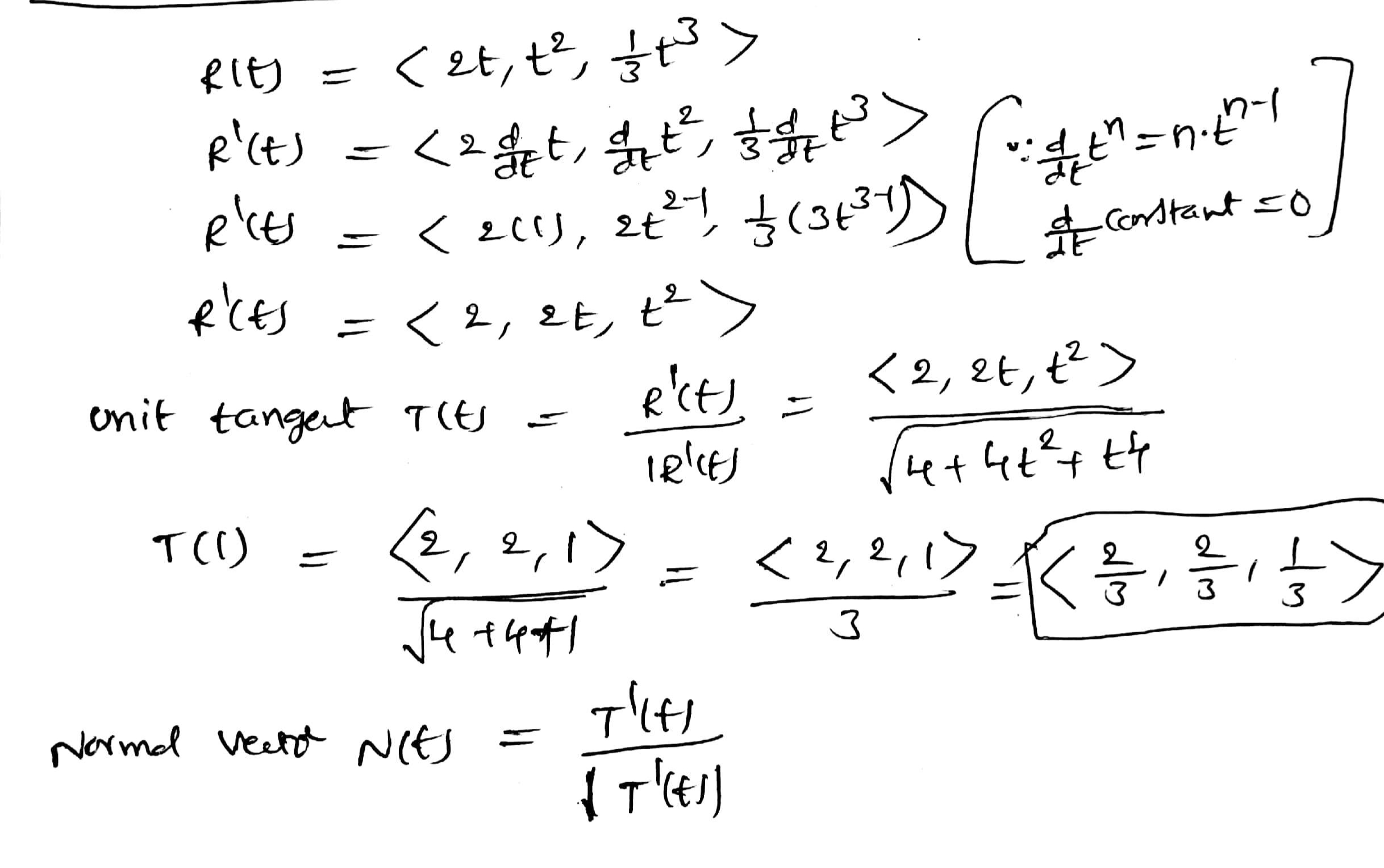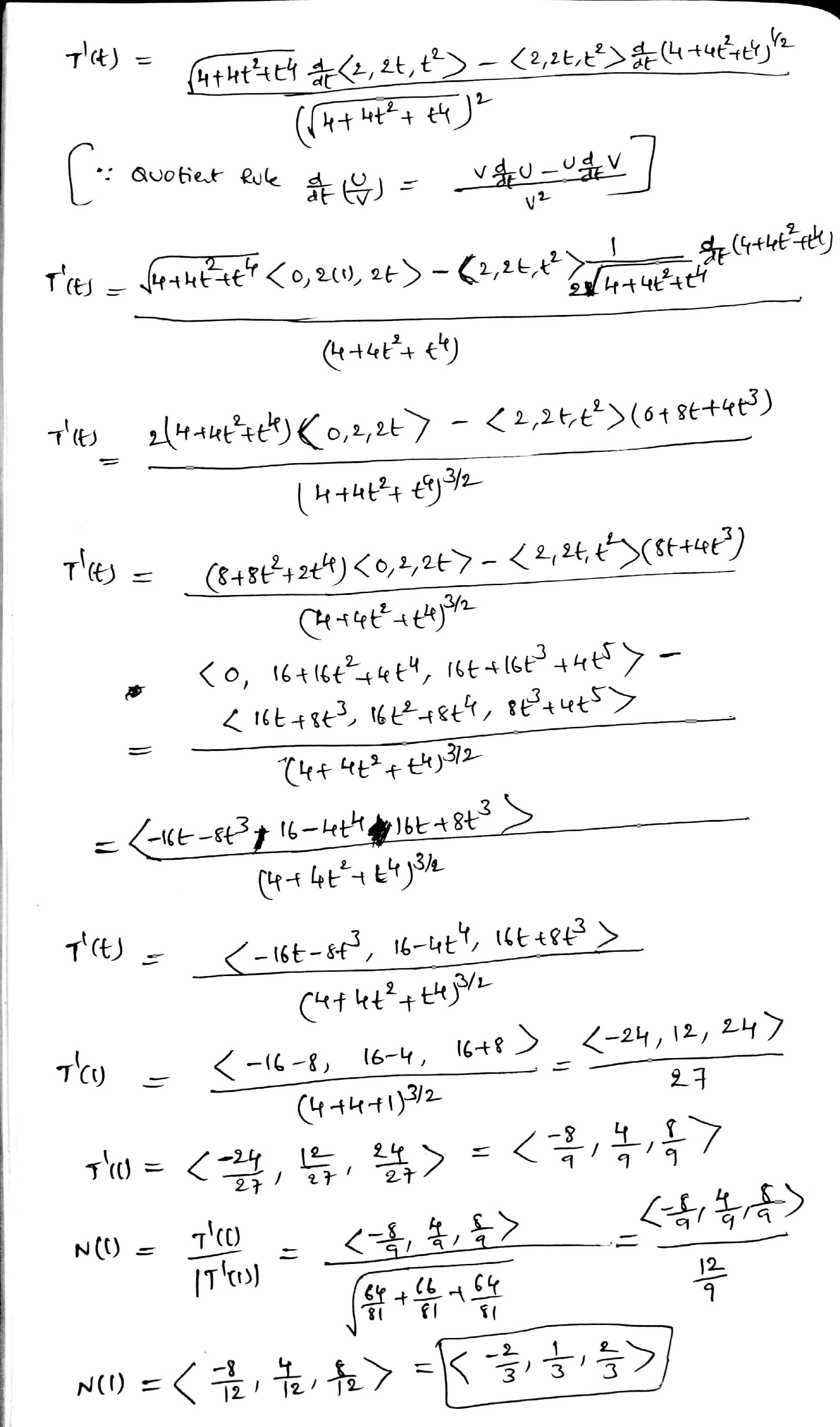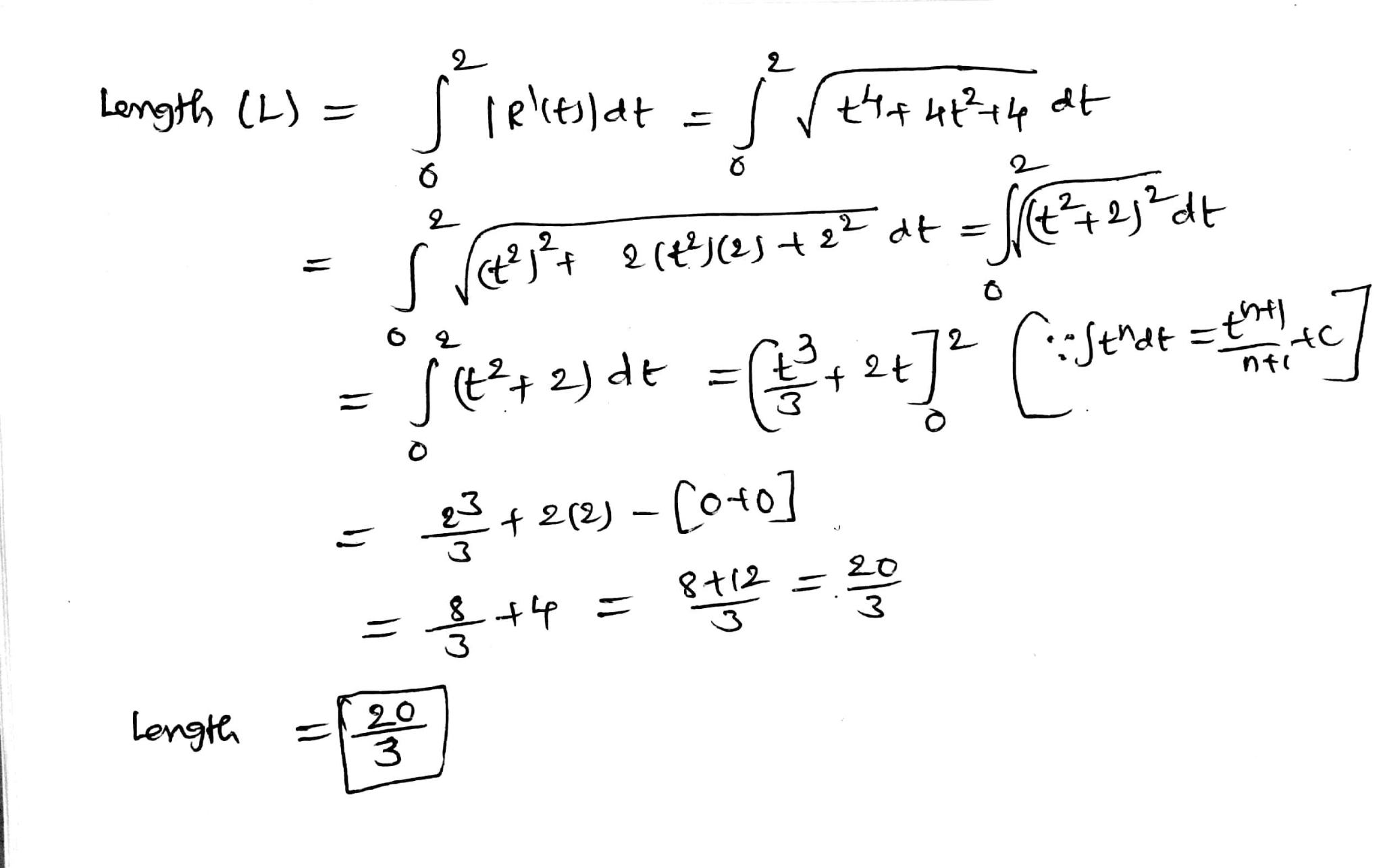In: Math

# compute the unit tangent vector T and the principal normal unit vector N of the space...

compute the unit tangent vector T and the principal normal unit vector N of the space curve R(t)=<2t, t^2, 1/3t^3> at the point when t=1. Then find its length over the domain [0,2]

## Solutions

##### Expert Solution## Related Solutions

##### Show the complete solution. Determine the unit tangent vector (T), the unit normal vector (N), and...
Show the complete solution. Determine the unit tangent vector (T), the unit normal vector (N), and the curvature of ?(?) = 2? ? + ?^2 ? – 1/3 ?^3 k at t = 1.
##### 15. a. Find the unit tangent vector T(1) at time t=1 for the space curve r(t)=〈t3...
15. a. Find the unit tangent vector T(1) at time t=1 for the space curve r(t)=〈t3 +3t, t2 +1, 3t+4〉. b. Compute the length of the space curve r(t) = 〈sin t, t, cos t〉 with 0 ≤ t ≤ 6.
##### (1 point) For the given position vectors r(t)r(t) compute the unit tangent vector T(t)T(t) for the...
(1 point) For the given position vectors r(t)r(t) compute the unit tangent vector T(t)T(t) for the given value of tt . A) Let r(t)=〈cos5t,sin5t〉 Then T(π4)〈 B) Let r(t)=〈t^2,t^3〉 Then T(4)=〈 C) Let r(t)=e^(5t)i+e^(−4t)j+tk Then T(−5)=
##### Consider the helix r(t)=(cos(2t),sin(2t),−3t)r(t)=(cos(2t),sin(2t),−3t). Compute, at t=π/6 A. The unit tangent vector T=T= ( , ,...
Consider the helix r(t)=(cos(2t),sin(2t),−3t)r(t)=(cos(2t),sin(2t),−3t). Compute, at t=π/6 A. The unit tangent vector T=T= ( , , ) B. The unit normal vector N=N= ( , , ) C. The unit binormal vector B=B= ( , , ) D. The curvature κ=κ=
##### Consider the following vector function. r(t) =<3t, 1/2 t2, t2> (a) Find the unit tangent and...
Consider the following vector function. r(t) =<3t, 1/2 t2, t2> (a) Find the unit tangent and unit normal vectors T(t) and N(t). (b). Find the curvature k(t).
##### FOR THE PARAMETRIZED PATH r(t)= e^tcos(πt)i+e^tsin(πt)j+e^tk a) find the velocity vector, the unit tangent vector and...
FOR THE PARAMETRIZED PATH r(t)= e^tcos(πt)i+e^tsin(πt)j+e^tk a) find the velocity vector, the unit tangent vector and the arc lenght between t=0 and t=1 b) find a point where the path given by r(t) intersects the plane x-y=0 and determine the angle of intersection between the tangent vector to the curve and the normal vector to the plane.
##### Compute the unit binormal vector and torsion of the curve: ?⃗⃗ (?) = 〈12?, 5cos ?,...
Compute the unit binormal vector and torsion of the curve: ?⃗⃗ (?) = 〈12?, 5cos ?, 5 sin ?〉
##### The motion of a particle in space is described by the vector equation ⃗r(t) = 〈sin...
The motion of a particle in space is described by the vector equation ⃗r(t) = 〈sin t, cos t, t〉 Identify the velocity and acceleration of the particle at (0,1,0) How far does the particle travel between t = 0 & t= pi What's the curvature of the particle at (0,1,0) & Find the tangential and normal components of the acceleration particle at (0,1,0)
##### Find T(t), N(t), aT, and aN at the given time t for the space curve r(t)....
Find T(t), N(t), aT, and aN at the given time t for the space curve r(t). [Hint: Find a(t), T(t), aT, and aN. Solve for N in the equation a(t)=aTT+aNN. (If an answer is undefined, enter UNDEFINED.) Function    Time r(t)=9ti-tj+(t^2)k t=-1 T(-1)= N(-1)= aT= aN=
##### Suppose time to failure follows normal distribution as, T tilde N open parentheses 2 comma space...
Suppose time to failure follows normal distribution as, T tilde N open parentheses 2 comma space 9 close parentheses. The failure rate function z(2.1) is